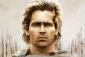# Arithmetic sequence

5 posts / 0 new
dan.and.yel
Arithmetic sequence

1/3, 1, 1/6, 2

is this an arithmetic sequence? i have seen it in a grade 10 book, thanks

Jhun VertNo it is not.

AlexanderArrange the numbers in increasing value: 1/6, 1/3, 1, 2. For a sequence to be arithmetic, the difference of two consecutive terms (any term - preceding term) must be equal. Let us check:

(a) 1/3 - 1/6 = 1/6
(b) 1 - 1/3 = 2/3
(c) 2 - 1 = 1

Since the difference of two adjacent terms are not equal, the given terms do not form into arithmetic sequence.

ケネス ケネスArithmetic sequence must be in increasing and deceasing value.

perfectveneer777

No. The sequence must be 1/3, 1/6, 1, 2.
a, a+d, a+2d, a+3d. Where a = 1/3, and d = 1/3.

• Mathematics inside the configured delimiters is rendered by MathJax. The default math delimiters are $$...$$ and $...$ for displayed mathematics, and $...$ and $...$ for in-line mathematics.